# Lions Weekly: August 24, 2022# A Note From Mr. Sandefur

Lion Family,

This week has been full of activity and excitement as the announcement of the J.T. Shen Science Center was made last week. This announcement paired with increasing enrollment has injected some energy into the campus that is encouraging. We humbly ask for your prayers as we manage the blessings that the Lord has provided as we move towards the goals that He has set ahead of us.

I wanted to take the opportunity this week to inform you of one additional change that is occurring this academic year. The Board of Trustees recently approved our leadership team to seek dual accreditation through the Association of Christian Schools International (ACSI) along with our current provider Cognia (formerly advancedEd/SACS). This move will add a layer of biblical integration standards to our already rigorous academic standards and aligns with our faith-based mission as an academic institution. We are currently completing the ACSI “Crosswalk” process where ACSI will become our accreditor this fall. Next spring (2024) we will go through our next full accreditation process with this specific change in place.

For those that are unaware, accreditation is the process in which an outside source ensures that The Academy is meeting and exceeding all standards for the education, safety, and well-being of its students. We are blessed to be a strongly accredited school and feel like this decision continues to place us on a path toward excellence in Christian education.

As always, we are grateful for your support and partnership in developing servant leaders that will change the world for Christ.

In Christ Alone,

Jeremy Sandefur

 table div table+table+table+table div table{width:100%;padding:0}table div table+table+table+table div table img{width:96.23%;padding:0;float:none}table div table+table+table+table div table td{width:100%;padding:0 1.88% 18px}/* styles */# Health Information

As we settle into a routine during this academic quarter, it is necessary to communicate effectively procedures regarding illness and attendance.

 table div table+table+table+table+table+table+table div table{width:100%;padding:0}table div table+table+table+table+table+table+table div table img{width:96.23%;padding:0;float:none}table div table+table+table+table+table+table+table div table td{width:100%;padding:0 1.88% 18px}/* styles */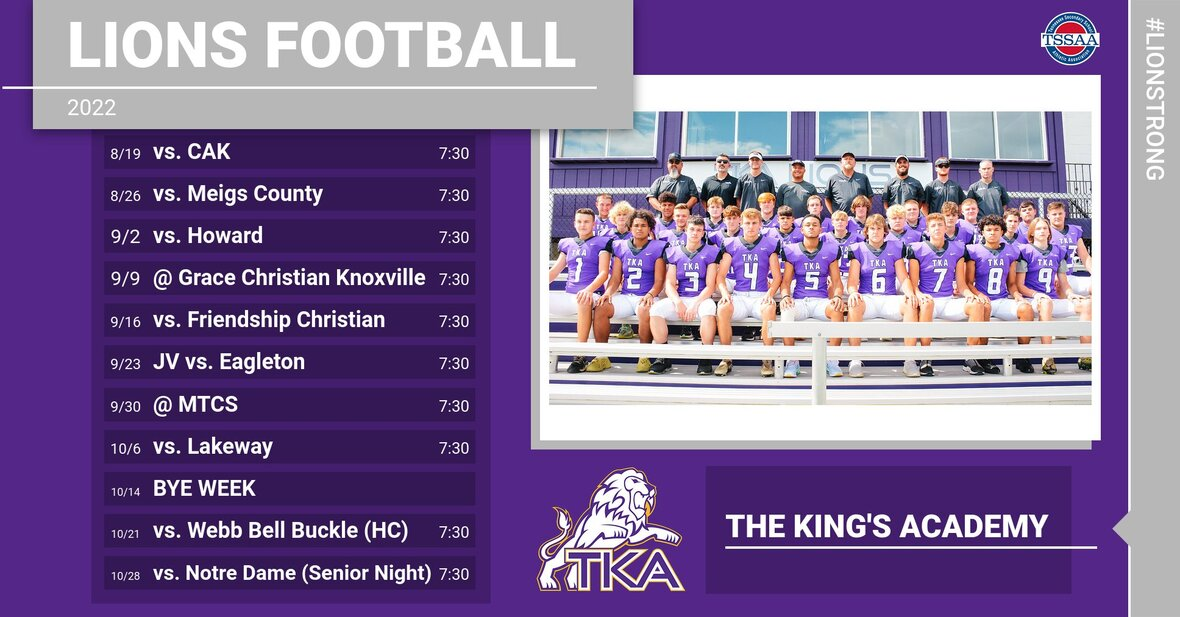# Athletic Event Tickets

This Friday is our second season football game! Mark your calendars and make plans to come cheer our Lions to victory! Below are a few updates for this season’s game tickets and parking passes:

▪ ALL TICKETS WILL BE SOLD ON GOFAN WE WILL NOT SELL PAPER TICKETS
▪ To purchase tickets on GoFan, use this link.
▪ All TKA students and staff members are free.
▪ All up-to-date TSSAA passes will be accepted.
▪ Individual season tickets are available for adults for \$60, students for \$35.
▪ Adult individual season tickets + parking pass available for \$95.
▪ Individual season parking passes available for \$40.
▪ Tickets are \$8 for adults, \$5 for students, kids 5 and under get in free.
▪ Parking is \$5 and parking passes are available on GoFan. If you buy your ticket through Go Fan, you may park in any of the four parking lots (Stokely (Pre-paid ONLY), Cartee (Pre-paid ONLY), Tibbetts, or Visitors). If you purchase parking upon arrival, the cost is \$5 but cash will ONLY be taken at the Tibbetts or Visitor lots.
▪ Free parking is available at Ogle and at the Weekly Softball Field.
▪ Gates will open at 6:00 p.m.
▪ Kickoff is at 7:30 p.m.
▪ Concessions will be available for purchase with cash or card.
 ▪ ALL TICKETS WILL BE SOLD ON GOFAN WE WILL NOT SELL PAPER TICKETS
 ▪ To purchase tickets on GoFan, use this link.
 ▪ All TKA students and staff members are free.
 ▪ All up-to-date TSSAA passes will be accepted.
 ▪ Individual season tickets are available for adults for \$60, students for \$35.
 ▪ Adult individual season tickets + parking pass available for \$95.
 ▪ Individual season parking passes available for \$40.
 ▪ Tickets are \$8 for adults, \$5 for students, kids 5 and under get in free.
 ▪ Parking is \$5 and parking passes are available on GoFan. If you buy your ticket through Go Fan, you may park in any of the four parking lots (Stokely (Pre-paid ONLY), Cartee (Pre-paid ONLY), Tibbetts, or Visitors). If you purchase parking upon arrival, the cost is \$5 but cash will ONLY be taken at the Tibbetts or Visitor lots.
 ▪ Free parking is available at Ogle and at the Weekly Softball Field.
 ▪ Gates will open at 6:00 p.m.
 ▪ Kickoff is at 7:30 p.m.
 ▪ Concessions will be available for purchase with cash or card.

See you Friday night at Huskey field!

 table div table+table+table+table+table+table+table+table+table+table div table{width:100%;padding:0}table div table+table+table+table+table+table+table+table+table+table div table img{width:96.23%;padding:0;float:none}table div table+table+table+table+table+table+table+table+table+table div table td{width:100%;padding:0 1.88% 18px}/* styles */# Dress Up Day: Surfing to Victory

This Friday, August 26, dress in your best beach/Hawaiian attire as we surf our way to victory!

Remember, all attire must adhere to dress code.

# Fall 2022 Dress Up Day Themes

Remember, all attire must adhere to dress code

▪ Friday, September 2 - Constructing a Championship Season (Dress in construction attire)
▪ Friday, September 16 - Hunting for a Repeat (Dress in Camo)
▪ Friday, September 23 - Gold Out (Dress in Gold for Childhood Cancer Awareness)
▪ Friday, October 7 - Pink Out (Dress in Pink for Breast Cancer Awareness)
▪ Tuesday, October 18 - Friday, October 21 - Homecoming Week (see details below)
▪ Friday, October 28 - "Senior" Spirit Day (Dress in "Senior" clothes in honor of Senior night)
 ▪ Friday, September 2 - Constructing a Championship Season (Dress in construction attire)
 ▪ Friday, September 16 - Hunting for a Repeat (Dress in Camo)
 ▪ Friday, September 23 - Gold Out (Dress in Gold for Childhood Cancer Awareness)
 ▪ Friday, October 7 - Pink Out (Dress in Pink for Breast Cancer Awareness)
 ▪ Tuesday, October 18 - Friday, October 21 - Homecoming Week (see details below)
 ▪ Friday, October 28 - "Senior" Spirit Day (Dress in "Senior" clothes in honor of Senior night)

## Homecoming Week: Blast from the Past

▪ Tuesday, October 18 - 90s Dress Up Day (Dress in 90s attire from movies, TV shows, musicians, or sports stars)
▪ Wednesday, October 19 - 80s Dress Up Day (Dress in 80s attire from movies, TV shows, musicians, or sports stars)
▪ Thursday, October 20 - 70s Dress Up Day (Dress in 70s attire from movies, TV shows, musicians, or sports stars)
▪ Friday, October 21 - Homecoming T-shirt or TKA Spirit attire
 ▪ Tuesday, October 18 - 90s Dress Up Day (Dress in 90s attire from movies, TV shows, musicians, or sports stars)
 ▪ Wednesday, October 19 - 80s Dress Up Day (Dress in 80s attire from movies, TV shows, musicians, or sports stars)
 ▪ Thursday, October 20 - 70s Dress Up Day (Dress in 70s attire from movies, TV shows, musicians, or sports stars)
 ▪ Friday, October 21 - Homecoming T-shirt or TKA Spirit attire
 table div table+table+table+table+table+table+table+table+table+table+table+table+table+table div table{width:100%;padding:0}table div table+table+table+table+table+table+table+table+table+table+table+table+table+table div table img{width:96.23%;padding:0;float:none}table div table+table+table+table+table+table+table+table+table+table+table+table+table+table div table td{width:100%;padding:0 1.88% 18px}/* styles */# Mum Sale

PTF will be selling 12" purple and yellow mums for \$18 each from now until August 31.

The girl or boy with the most mum sales will be the crown and ball holder at the Homecoming game on Friday, October 21.

 table div table+table+table+table+table+table+table+table+table+table+table+table+table+table+table+table+table div table{width:100%;padding:0}table div table+table+table+table+table+table+table+table+table+table+table+table+table+table+table+table+table div table img{width:96.23%;padding:0;float:none}table div table+table+table+table+table+table+table+table+table+table+table+table+table+table+table+table+table div table td{width:100%;padding:0 1.88% 18px}/* styles */# Daily Announcements

## Elementary

Did you know that you can listen to Ms. Sullivan's weekly announcements? Open the TKA app and go to "elementary". Then, click "link list" followed by clicking on "TKA Elementary Site by Principal Sullivan". There you will find a link to Ms. Sullivan's announcements under the Weekly Announcement tab.

## Middle and High

Did you know that you can listen to Mr. Fuller's morning announcements in the app? Simply open the app and go to "watch". There you will find a link to Mr. Fuller's daily announcements for middle and high schoolers.

 table div table+table+table+table+table+table+table+table+table+table+table+table+table+table+table+table+table+table+table div table{width:100%;padding:0}table div table+table+table+table+table+table+table+table+table+table+table+table+table+table+table+table+table+table+table div table img{width:96.23%;padding:0;float:none}table div table+table+table+table+table+table+table+table+table+table+table+table+table+table+table+table+table+table+table div table td{width:100%;padding:0 1.88% 18px}/* styles */# Elementary Fall Clubs

TKA Elementary Families,

We are excited to announce that our TKA Elementary club opportunities will begin very soon. Each club will have a fall section and a spring section.

The fall section will begin the week of September 12th and end December 9th. The spring section will begin the week of January 30th and end April 21st. Registration for the spring sections will be shared second semester.

Each club will include a \$20 participation fee to cover resources needed for club activities. Each club has a maximum number of 15 students.

Please click on a club to review the club description and registration link. We will select the first participants until the maximum number is reached. We ask that you consider all options and register for clubs that fit your schedule for the year. To be fair to our club instructors, we ask that you choose a club where your child will be able to participate and attend 90% of the semester club activities.

All sign up forms will close Friday, September 2nd. Our club teachers will be in communication with participant families for their club area by Friday, September 9th. This communication will include the next steps prior to the first meeting the following week.

We are very blessed to have such wonderful teachers who are excited to provide these enriching opportunities after school hours to further enhance our elementary learning.

Blessings,
Kelly Sullivan
Elementary Principal

 table div table+table+table+table+table+table+table+table+table+table+table+table+table+table+table+table+table+table+table+table+table div table{width:100%;padding:0}table div table+table+table+table+table+table+table+table+table+table+table+table+table+table+table+table+table+table+table+table+table div table img{width:96.23%;padding:0;float:none}table div table+table+table+table+table+table+table+table+table+table+table+table+table+table+table+table+table+table+table+table+table div table td{width:100%;padding:0 1.88% 18px}/* styles */Book Club will provide students with the opportunity to engage with texts deeply in a relaxed fun environment. Through group discussion and hands-on activities, students will practice age appropriate comprehension skills such as character analysis, distinguishing literary themes, understanding the elements of plot development, and using textual evidence. Book club will also provide time for readers to share book recommendations with each other, through book talks and book lists, in order to deepen their self-knowledge as a reader and broaden their range of choice in reading material. Reading choice is vital to developing a lifelong love of reading. Choice of club texts will be determined in the first few meetings of the club so readers can have input and ownership of their experience.

 table div table+table+table+table+table+table+table+table+table+table+table+table+table+table+table+table+table+table+table+table+table+table+table+table+table div table{width:100%;padding:0}table div table+table+table+table+table+table+table+table+table+table+table+table+table+table+table+table+table+table+table+table+table+table+table+table+table div table img{width:96.23%;padding:0;float:none}table div table+table+table+table+table+table+table+table+table+table+table+table+table+table+table+table+table+table+table+table+table+table+table+table+table div table td{width:100%;padding:0 1.88% 18px}/* styles */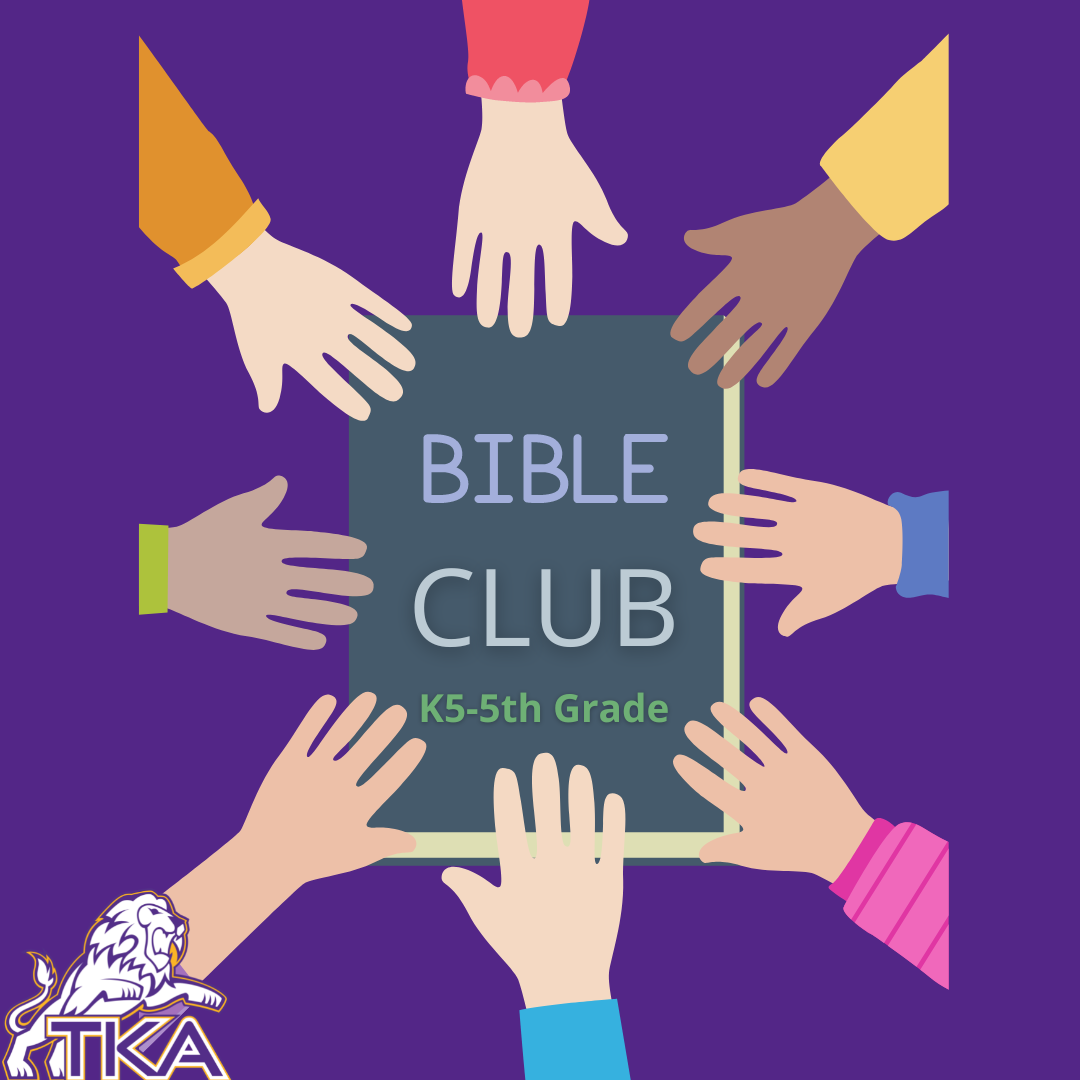Bible Club will include Bible Drill, Memory Verses, Bible Story Interacting (drawing, drama, games, questions, songs,) . . . and Developing a Personal Quiet Time.

 table div table+table+table+table+table+table+table+table+table+table+table+table+table+table+table+table+table+table+table+table+table+table+table+table+table+table+table+table+table div table{width:100%;padding:0}table div table+table+table+table+table+table+table+table+table+table+table+table+table+table+table+table+table+table+table+table+table+table+table+table+table+table+table+table+table div table img{width:96.23%;padding:0;float:none}table div table+table+table+table+table+table+table+table+table+table+table+table+table+table+table+table+table+table+table+table+table+table+table+table+table+table+table+table+table div table td{width:100%;padding:0 1.88% 18px}/* styles */# K4-K5 STEAM Club

K4-K5 STEAM Club will include a variety of hands-on activities focusing on science, technology, engineering, arts, and mathematics that are age appropriate for our little lions.

 table div table+table+table+table+table+table+table+table+table+table+table+table+table+table+table+table+table+table+table+table+table+table+table+table+table+table+table+table+table+table+table+table div table{width:100%;padding:0}table div table+table+table+table+table+table+table+table+table+table+table+table+table+table+table+table+table+table+table+table+table+table+table+table+table+table+table+table+table+table+table+table div table img{width:96.23%;padding:0;float:none}table div table+table+table+table+table+table+table+table+table+table+table+table+table+table+table+table+table+table+table+table+table+table+table+table+table+table+table+table+table+table+table+table div table td{width:100%;padding:0 1.88% 18px}/* styles */# STEAM Club (1-2 grades only)

This STEAM Club will include a variety of hands-on activities focusing on science, technology, engineering, arts, and mathematics that are age appropriate for our primary lions.

 table div table+table+table+table+table+table+table+table+table+table+table+table+table+table+table+table+table+table+table+table+table+table+table+table+table+table+table+table+table+table+table+table+table+table+table div table{width:100%;padding:0}table div table+table+table+table+table+table+table+table+table+table+table+table+table+table+table+table+table+table+table+table+table+table+table+table+table+table+table+table+table+table+table+table+table+table+table div table img{width:96.23%;padding:0;float:none}table div table+table+table+table+table+table+table+table+table+table+table+table+table+table+table+table+table+table+table+table+table+table+table+table+table+table+table+table+table+table+table+table+table+table+table div table td{width:100%;padding:0 1.88% 18px}/* styles */# Whiz Kidz (3-5 grades only)

This STEAM Club will include a variety of hands-on activities focusing on science, technology, engineering, arts, and mathematics that are age appropriate for our intermediate lions.

 table div table+table+table+table+table+table+table+table+table+table+table+table+table+table+table+table+table+table+table+table+table+table+table+table+table+table+table+table+table+table+table+table+table+table+table+table+table+table div table{width:100%;padding:0}table div table+table+table+table+table+table+table+table+table+table+table+table+table+table+table+table+table+table+table+table+table+table+table+table+table+table+table+table+table+table+table+table+table+table+table+table+table+table div table img{width:96.23%;padding:0;float:none}table div table+table+table+table+table+table+table+table+table+table+table+table+table+table+table+table+table+table+table+table+table+table+table+table+table+table+table+table+table+table+table+table+table+table+table+table+table+table div table td{width:100%;padding:0 1.88% 18px}/* styles */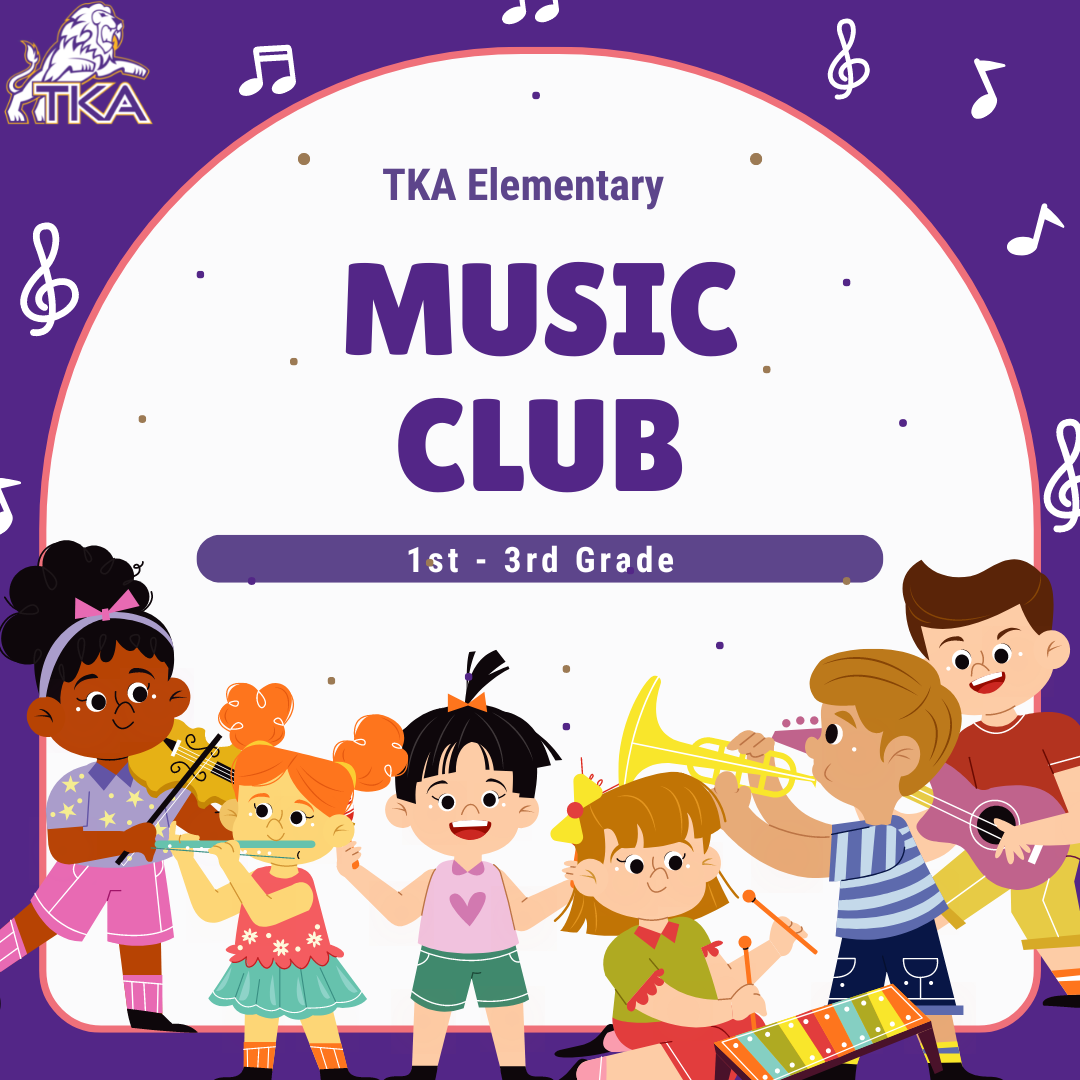# Music Club (Grades 1-3 only)

Join us as we Create, Perform, and Respond to music in lots of fun ways! A few of the activities we will be doing:

▪ Singing
▪ Playing instruments
▪ Moving to music
▪ Listening to music
▪ Composing, arranging, and performing music
 ▪ Singing
 ▪ Playing instruments
 ▪ Moving to music
 ▪ Listening to music
 ▪ Composing, arranging, and performing music
 table div table+table+table+table+table+table+table+table+table+table+table+table+table+table+table+table+table+table+table+table+table+table+table+table+table+table+table+table+table+table+table+table+table+table+table+table+table+table+table+table+table div table{width:100%;padding:0}table div table+table+table+table+table+table+table+table+table+table+table+table+table+table+table+table+table+table+table+table+table+table+table+table+table+table+table+table+table+table+table+table+table+table+table+table+table+table+table+table+table div table img{width:96.23%;padding:0;float:none}table div table+table+table+table+table+table+table+table+table+table+table+table+table+table+table+table+table+table+table+table+table+table+table+table+table+table+table+table+table+table+table+table+table+table+table+table+table+table+table+table+table div table td{width:100%;padding:0 1.88% 18px}/* styles */# 2021-22 Yearbooks

Preordered yearbooks, are in and delivered to students. If you did not order a yearbook for 2021-22, they can be purchased for \$55 in either school office or by emailing Mr. Josh Smith jsmith@thekingsacademy.net.

 table div table+table+table+table+table+table+table+table+table+table+table+table+table+table+table+table+table+table+table+table+table+table+table+table+table+table+table+table+table+table+table+table+table+table+table+table+table+table+table+table+table+table+table div table{width:100%;padding:0}table div table+table+table+table+table+table+table+table+table+table+table+table+table+table+table+table+table+table+table+table+table+table+table+table+table+table+table+table+table+table+table+table+table+table+table+table+table+table+table+table+table+table+table div table img{width:96.23%;padding:0;float:none}table div table+table+table+table+table+table+table+table+table+table+table+table+table+table+table+table+table+table+table+table+table+table+table+table+table+table+table+table+table+table+table+table+table+table+table+table+table+table+table+table+table+table+table div table td{width:100%;padding:0 1.88% 18px}/* styles */# 9-12th Spring Break Trip: France, Germany and The Alps

## March 11-18, 2023

 table div table+table+table+table+table+table+table+table+table+table+table+table+table+table+table+table+table+table+table+table+table+table+table+table+table+table+table+table+table+table+table+table+table+table+table+table+table+table+table+table+table+table+table+table+table div table{width:100%;padding:0}table div table+table+table+table+table+table+table+table+table+table+table+table+table+table+table+table+table+table+table+table+table+table+table+table+table+table+table+table+table+table+table+table+table+table+table+table+table+table+table+table+table+table+table+table+table div table img{width:96.23%;padding:0;float:none}table div table+table+table+table+table+table+table+table+table+table+table+table+table+table+table+table+table+table+table+table+table+table+table+table+table+table+table+table+table+table+table+table+table+table+table+table+table+table+table+table+table+table+table+table+table div table td{width:100%;padding:0 1.88% 18px}/* styles */# Weekly Sports Schedule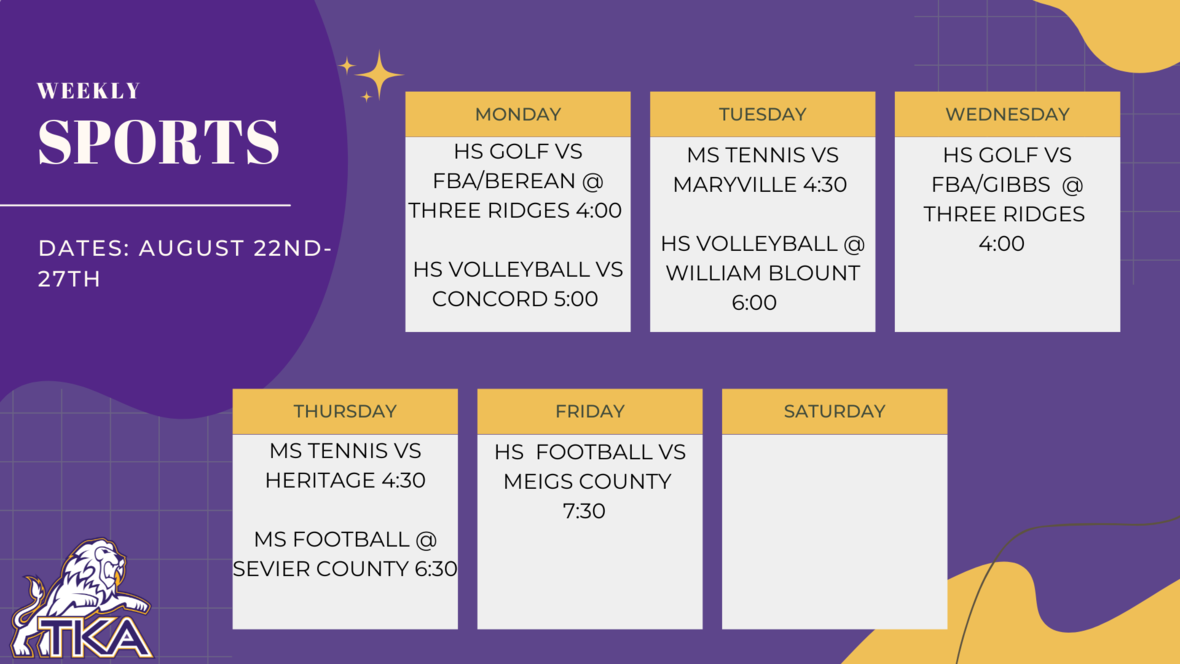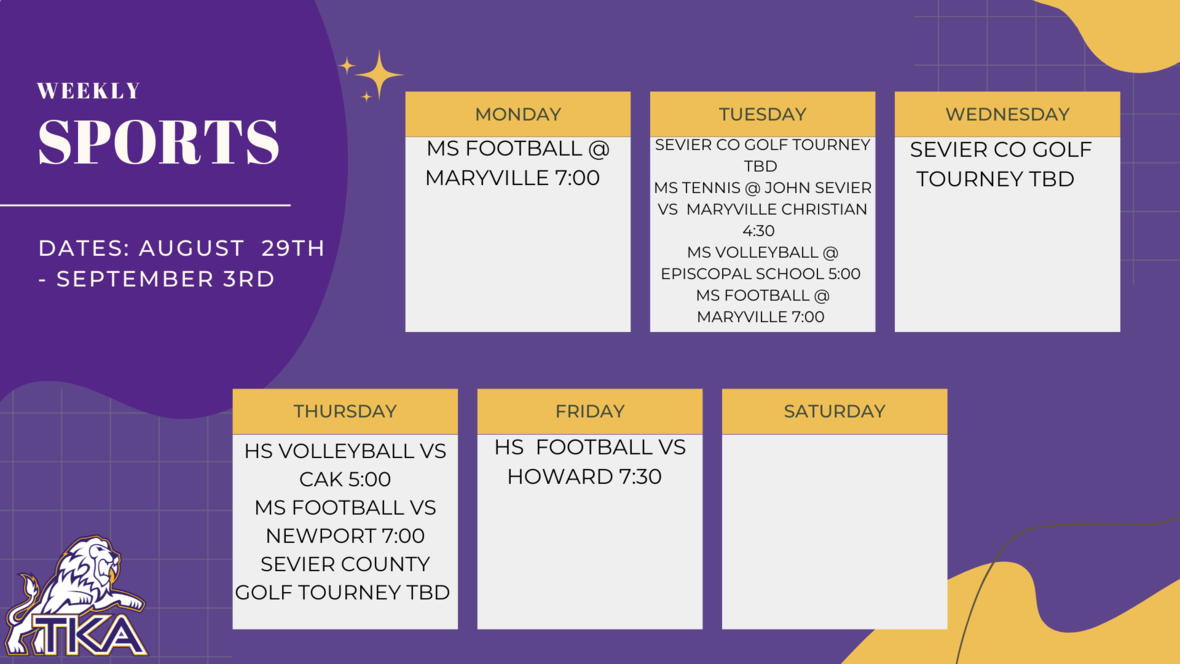table div table+table+table+table+table+table+table+table+table+table+table+table+table+table+table+table+table+table+table+table+table+table+table+table+table+table+table+table+table+table+table+table+table+table+table+table+table+table+table+table+table+table+table+table+table+table+table+table+table div table{width:100%;padding:0}table div table+table+table+table+table+table+table+table+table+table+table+table+table+table+table+table+table+table+table+table+table+table+table+table+table+table+table+table+table+table+table+table+table+table+table+table+table+table+table+table+table+table+table+table+table+table+table+table+table div table img{width:96.23%;padding:0;float:none}table div table+table+table+table+table+table+table+table+table+table+table+table+table+table+table+table+table+table+table+table+table+table+table+table+table+table+table+table+table+table+table+table+table+table+table+table+table+table+table+table+table+table+table+table+table+table+table+table+table div table td{width:100%;padding:0 1.88% 18px}/* styles */# Important information for the 2022-2023 School Year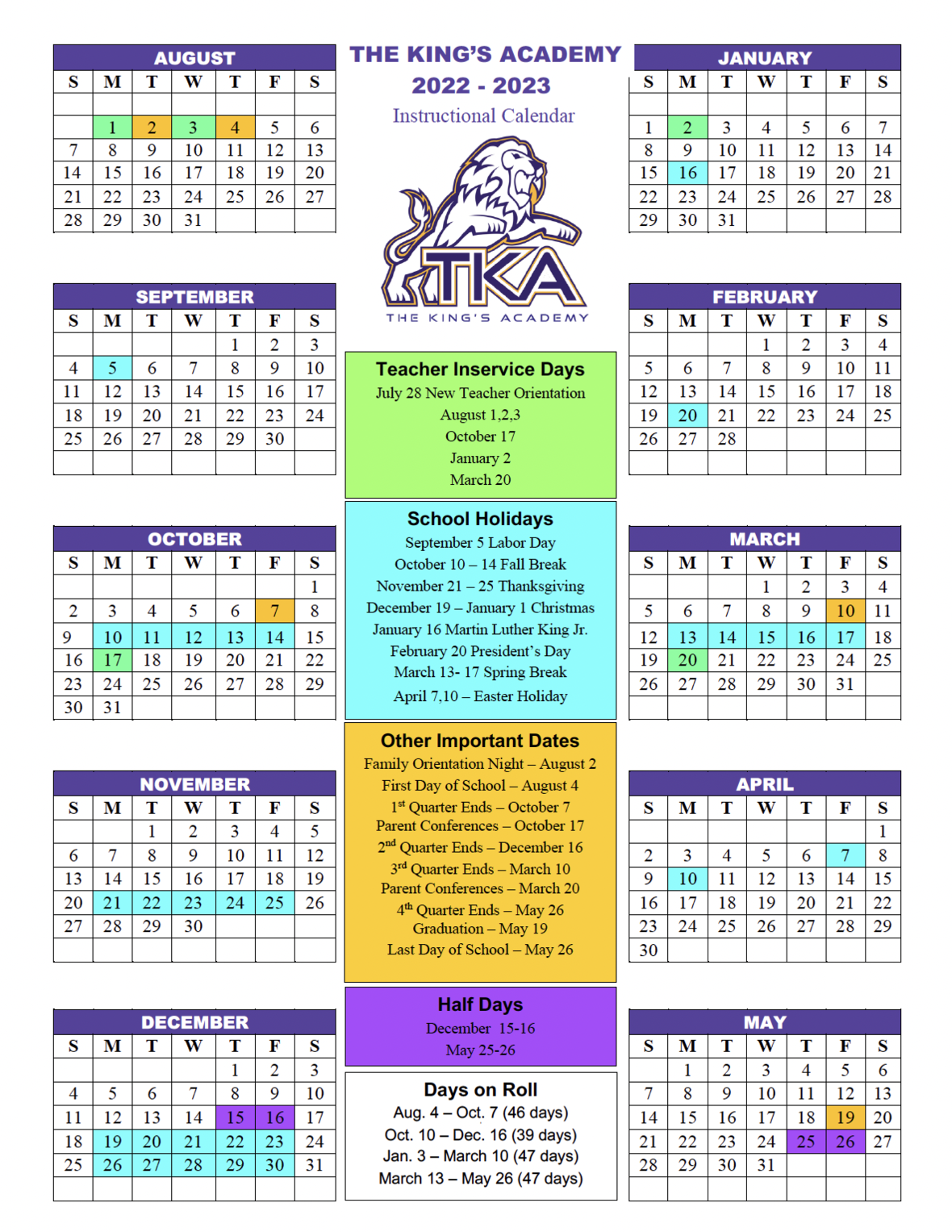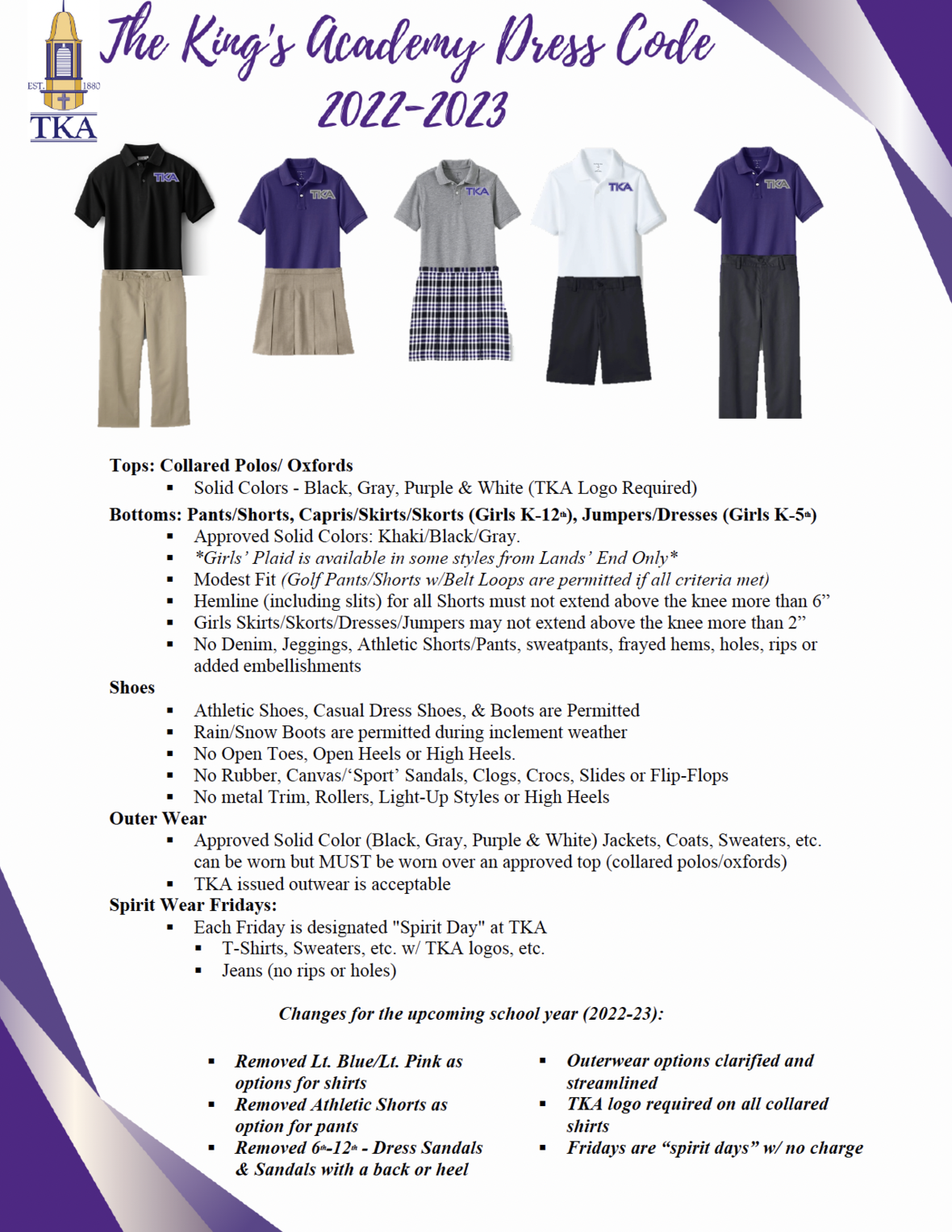table div table+table+table+table+table+table+table+table+table+table+table+table+table+table+table+table+table+table+table+table+table+table+table+table+table+table+table+table+table+table+table+table+table+table+table+table+table+table+table+table+table+table+table+table+table+table+table+table+table+table+table+table+table div table td,table.module-52{width:100%;padding:0}table div table+table+table+table+table+table+table+table+table+table+table+table+table+table+table+table+table+table+table+table+table+table+table+table+table+table+table+table+table+table+table+table+table+table+table+table+table+table+table+table+table+table+table+table+table+table+table+table+table+table+table+table+table div table{width:100%;float:none;margin-left:auto;margin-right:auto;padding:0}table div table+table+table+table+table+table+table+table+table+table+table+table+table+table+table+table+table+table+table+table+table+table+table+table+table+table+table+table+table+table+table+table+table+table+table+table+table+table+table+table+table+table+table+table+table+table+table+table+table+table+table+table+table div table a{border:0 none;text-decoration:none}table div table+table+table+table+table+table+table+table+table+table+table+table+table+table+table+table+table+table+table+table+table+table+table+table+table+table+table+table+table+table+table+table+table+table+table+table+table+table+table+table+table+table+table+table+table+table+table+table+table+table+table+table+table div table img{width:100%!important;border:0 none;text-decoration:none}/* styles */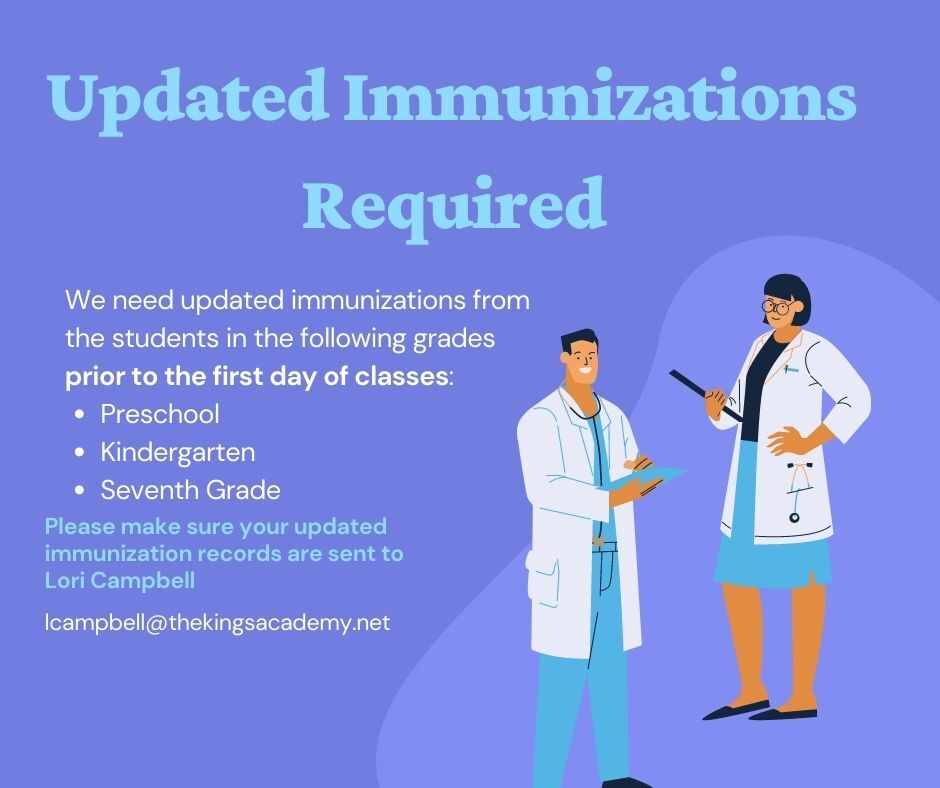table div table+table+table+table+table+table+table+table+table+table+table+table+table+table+table+table+table+table+table+table+table+table+table+table+table+table+table+table+table+table+table+table+table+table+table+table+table+table+table+table+table+table+table+table+table+table+table+table+table+table+table+table+table+table+table div table{width:100%;padding:0}table div table+table+table+table+table+table+table+table+table+table+table+table+table+table+table+table+table+table+table+table+table+table+table+table+table+table+table+table+table+table+table+table+table+table+table+table+table+table+table+table+table+table+table+table+table+table+table+table+table+table+table+table+table+table+table div table img{width:96.23%;padding:0;float:none}table div table+table+table+table+table+table+table+table+table+table+table+table+table+table+table+table+table+table+table+table+table+table+table+table+table+table+table+table+table+table+table+table+table+table+table+table+table+table+table+table+table+table+table+table+table+table+table+table+table+table+table+table+table+table+table div table td{width:100%;padding:0 1.88% 18px}/* styles */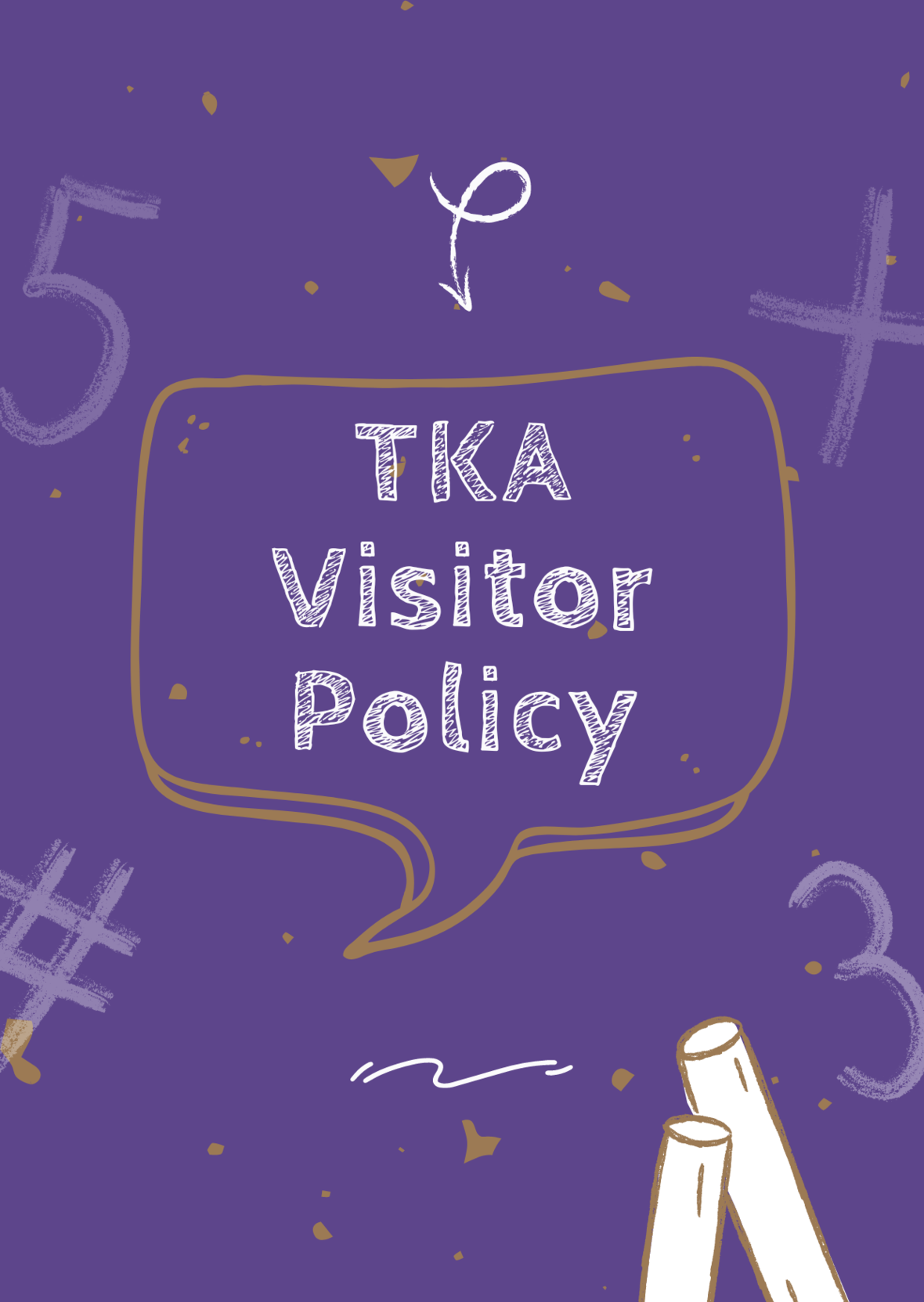# TKA Visitor Policy

In order to help maintain a safe and instructionally based learning environment for our students, all visitors for middle and high school must enter through the front door of the Anderson Building and sign in in the middle/high school office in order to receive a visitor's pass. All visitors for elementary must enter the front door of the Ogle Building in order to receive a visitor's pass. This pass should be visible at all times so that TKA faculty and staff know that you have checked in at the office.

Our doors are open to visitors, but please call ahead of time to schedule conferences with your child’s teachers. This will prevent interruptions to instruction and the loss of the valuable learning time of our students. Adults will not be allowed to wander the halls of the building or hold conferences with teachers during instructional times.

TKA family lunch visitors should follow the above procedure. Lunch tickets are \$5 each.

Students who are not enrolled at TKA are not permitted to visit TKA students during the school day, which includes lunch.

This policy has been developed in order to help maintain a safe and instruction focused environment at school each day. Any visitor who does not meet these expectations may be asked to leave school grounds.

 table div table+table+table+table+table+table+table+table+table+table+table+table+table+table+table+table+table+table+table+table+table+table+table+table+table+table+table+table+table+table+table+table+table+table+table+table+table+table+table+table+table+table+table+table+table+table+table+table+table+table+table+table+table+table+table+table+table div table{width:100%;padding:0}table div table+table+table+table+table+table+table+table+table+table+table+table+table+table+table+table+table+table+table+table+table+table+table+table+table+table+table+table+table+table+table+table+table+table+table+table+table+table+table+table+table+table+table+table+table+table+table+table+table+table+table+table+table+table+table+table+table div table img{width:96.23%;padding:0;float:none}table div table+table+table+table+table+table+table+table+table+table+table+table+table+table+table+table+table+table+table+table+table+table+table+table+table+table+table+table+table+table+table+table+table+table+table+table+table+table+table+table+table+table+table+table+table+table+table+table+table+table+table+table+table+table+table+table+table div table td{width:100%;padding:0 1.88% 18px}/* styles *//* styles */
 table div table+table+table+table+table+table+table+table+table+table+table+table+table+table+table+table+table+table+table+table+table+table+table+table+table+table+table+table+table+table+table+table+table+table+table+table+table+table+table+table+table+table+table+table+table+table+table+table+table+table+table+table+table+table+table+table+table+table+table+table div table{width:100%;padding:0}table div table+table+table+table+table+table+table+table+table+table+table+table+table+table+table+table+table+table+table+table+table+table+table+table+table+table+table+table+table+table+table+table+table+table+table+table+table+table+table+table+table+table+table+table+table+table+table+table+table+table+table+table+table+table+table+table+table+table+table+table div table img{width:96.23%;padding:0;float:none}table div table+table+table+table+table+table+table+table+table+table+table+table+table+table+table+table+table+table+table+table+table+table+table+table+table+table+table+table+table+table+table+table+table+table+table+table+table+table+table+table+table+table+table+table+table+table+table+table+table+table+table+table+table+table+table+table+table+table+table+table div table td{width:100%;padding:0 1.88% 18px}/* styles */table div table+table+table+table+table+table+table+table+table+table+table+table+table+table+table+table+table+table+table+table+table+table+table+table+table+table+table+table+table+table+table+table+table+table+table+table+table+table+table+table+table+table+table+table+table+table+table+table+table+table+table+table+table+table+table+table+table+table+table+table+table+table div table{width:100%;padding:0}table div table+table+table+table+table+table+table+table+table+table+table+table+table+table+table+table+table+table+table+table+table+table+table+table+table+table+table+table+table+table+table+table+table+table+table+table+table+table+table+table+table+table+table+table+table+table+table+table+table+table+table+table+table+table+table+table+table+table+table+table+table+table div table img{width:96.23%;padding:0;float:none}table div table+table+table+table+table+table+table+table+table+table+table+table+table+table+table+table+table+table+table+table+table+table+table+table+table+table+table+table+table+table+table+table+table+table+table+table+table+table+table+table+table+table+table+table+table+table+table+table+table+table+table+table+table+table+table+table+table+table+table+table+table+table div table td{width:100%;padding:0 1.88% 18px}/* styles *//* styles */ The King's Academy
 Like   Tweet